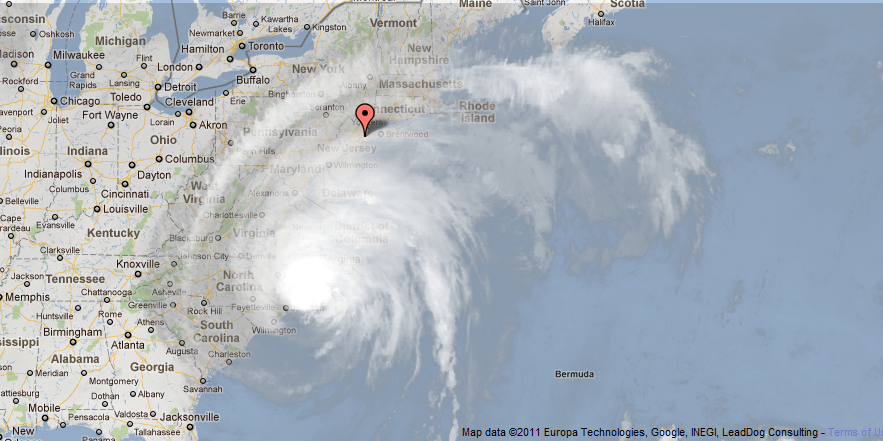# Hurricane Irene – Logarithmic Spiral?

Does Hurricane Irene fit a logarithmic spiral?I made a Geogebra applet to see if it does. Enjoy.

Parametric equation:$x=b*cos(t)*e^{at}$$y=b*sin(t)*e^{at}$$-11.7\le t \le 3.4$